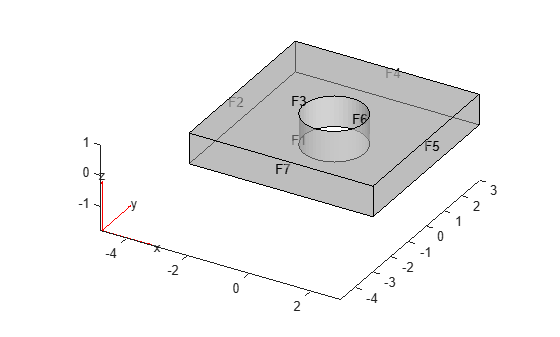# Geometry from `alphaShape`

Create a 3-D geometry using the MATLAB `alphaShape` function. First, create an `alphaShape` object of a block with a cylindrical hole. Then import the geometry into a PDE model from the `alphaShape` boundary.

Create a 2-D mesh grid.

```[xg,yg] = meshgrid(-3:0.25:3); xg = xg(:); yg = yg(:);```

Create a unit disk. Remove all the mesh grid points that fall inside the unit disk, and include the unit disk points.

```t = (pi/24:pi/24:2*pi)'; x = cos(t); y = sin(t); circShp = alphaShape(x,y,2); in = inShape(circShp,xg,yg); xg = [xg(~in); cos(t)]; yg = [yg(~in); sin(t)];```

Create 3-D copies of the remaining mesh grid points, with the `z`-coordinates ranging from 0 through 1. Combine the points into an `alphaShape` object.

```zg = ones(numel(xg),1); xg = repmat(xg,5,1); yg = repmat(yg,5,1); zg = zg*(0:.25:1); zg = zg(:); shp = alphaShape(xg,yg,zg);```

Obtain a surface mesh of the `alphaShape` object.

`[elements,nodes] = boundaryFacets(shp);`

Put the data in the correct shape for `geometryFromMesh`.

```nodes = nodes'; elements = elements';```

Create a PDE model and import the surface mesh.

```model = createpde(); geometryFromMesh(model,nodes,elements);```

View the geometry and face numbers.

`pdegplot(model,'FaceLabels','on','FaceAlpha',0.5)`To use the geometry in an analysis, create a volume mesh.

`generateMesh(model);`

## SupportGet trial now